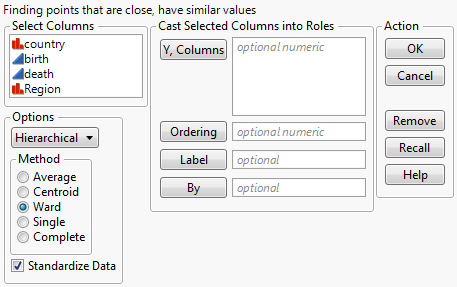Scripting Guide > Display Trees > Construct Custom Windows > Example of Creating a Cluster Platform Launch Window
Publication date: 03/23/2021

## Example of Creating a Cluster Platform Launch Window

The following example creates a simplified replica of the Cluster platform launch window, which then launches the platform with the given arguments.

Note: Some functions (Recall and Help) are not implemented in the script, so an alert window is shown when the Recall and Help button are clicked. In addition, switching from Hierarchical to K-Means clustering does not change anything, unlike the real Cluster launch window.

`dt = Open( "\$SAMPLE_DATA/Birth Death.jmp" );`
`nc = N Col( dt ); // number of columns in the window`
`lbWidth = 130; // width of the window`
` `

// define the method list

`methodList = {"Average", "Centroid", "Ward", "Single", "Complete"};`
`notImplemented = Expr(`
`	win = New Window( "Feature Not Implemented Yet", <<Modal, Button Box( "OK" ) )`
`);`
`clusterDlg = New Window( "Clustering", // create the window`
`	<<Modal,`
`	Border Box( Left( 3 ), Top( 2 ),`
`		V List Box(`
`			Text Box( "Finding points that are close, have similar values" ),`
`			H List Box(`
`				V List Box(`
`					Panel Box( "Select Columns",`
`						colListData = Col List Box(`
`							All,`
`							width( lbWidth ),`
`							NLines( Min( nc, 10 ) )`
`						)`
`					),`
`					Panel Box( "Options",`
`						V List Box(`
`							comboObj = Combo Box(`
`								{"Hierarchical", "K-Means"},`
`								<<Set( 1 )`
`							),`
`							Panel Box( "Method",`
`								methodObj = Radio Box( methodList, <<Set( 3 ) )`
`							),`
`							checkObj = Check Box( {"Standardize Data"}, <<Set( 1, 1 ) )`
`						)`
`					)`
`				),`
`				Panel Box( "Cast Selected Columns into Roles",`
`					Line Up Box( N Col( 2 ), Spacing( 3 ),`
`						Button Box( "Y, Columns",`
`							colListY << Append( colListData << GetSelected )`
`						),`
`						colListY = Col List Box(`
`							width( lbWidth ),`
`							NLines( 5 ),`
`							"numeric"`
`						),`
`						Button Box( "Ordering",`
`							colListO << Append( colListData << GetSelected )`
`						),`
`						colListO = Col List Box(`
`							width( lbWidth ),`
`							NLines( 1 ),`
`							"numeric"`
`						),`
`						Button Box( "Label",`
`							colListL << Append( colListData << GetSelected )`
`						),`
`						colListL = Col List Box( width( lbWidth ), NLines( 1 ) ),`
`						Button Box( "By",`
`							colListB << Append( colListData << GetSelected )`
`						),`
`						colListB = Col List Box( width( lbWidth ), NLines( 1 ) )`
`					)`
`				),`
`				Panel Box( "Action",`
`					Line Up Box( N Col( 1 ),`
`						Button Box( "OK",`
`							If( (comboObj << Get) == 1,`
`								Hierarchical Cluster(`
`									Y( Eval( colListY << GetItems ) ),`
`									Order( Eval( colListO << GetItems ) ),`
`									Label( Eval( colListL << GetItems ) ),`
`									By( Eval( colListB << GetItems ) ),`
`									Method( methodList[methodObj << Get]`
`									),`
`									Standardize( checkObj << Get( 1 ) )`
`								),`
`								KMeansCluster( Y( colListY << GetItems ) )`
`							);`
`							clusterDlg << Close Window;`
`						),`
`						Button Box( "Cancel", clusterDlg << Close Window ),`
`						Text Box( " " ),`
`						Button Box( "Remove",`
`							colListY << RemoveSelected;`
`							colListO << RemoveSelected;`
`							colListL << RemoveSelected;`
`							colListB << RemoveSelected;`
`						),`
`						Button Box( "Recall", notImplemented ),`
`						Button Box( "Help", notImplemented )`
`					)`
`				)`
`			)`
`		)`
`	)`
`);`

Figure 11.31 The Cluster Launch WindowWant more information? Have questions? Get answers in the JMP User Community (community.jmp.com).